# Average value, theorem on variations of the

in statistical mechanics

A statement on the variation of the average value of a dynamic magnitude in the Gibbs statistical aggregate as a result of an infinitesimal change in the Hamiltonian. The variation of the average depends, generally speaking, on the way of "inclusion" of the change in the Hamiltonian and on the initial conditions. Let a system of many interacting particles (a quantum system or a classical system), that can explicitly be described by a time-independent Hamiltonian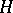, be in thermodynamic equilibrium at the initial moment of time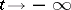. As a result of an adiabatic inclusion of an infinitesimal, time-dependent perturbation of the Hamiltonian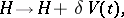where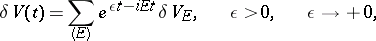the Gibbs equilibrium average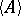of the explicitly time-independent dynamic variablewill change (in a linear approximation with respect to the perturbation) by the magnitude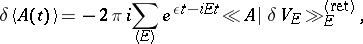where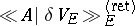is the Fourier transform of the retarded commutator Green function (cf. Green function in statistical mechanics).

The main application of the theorem is in the theory of non-equilibrium irreversible processes (in which one formulation of the theorem is also known as the fluctuation-dissipation theorem), and in deducing the chains and systems of equations for the Green functions from the chains and systems of equations for the correlation functions (cf. Correlation function in statistical mechanics).